Ex 4.1

Chapter 4 Class 11 Mathematical Induction
Serial order wise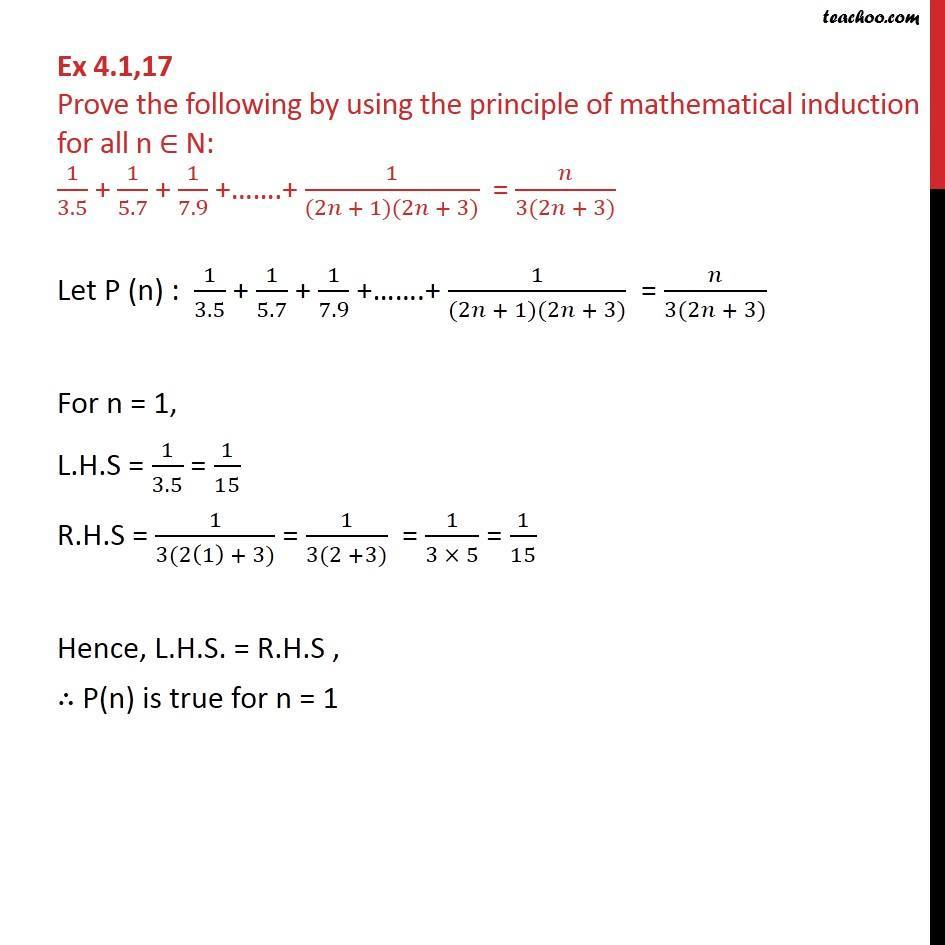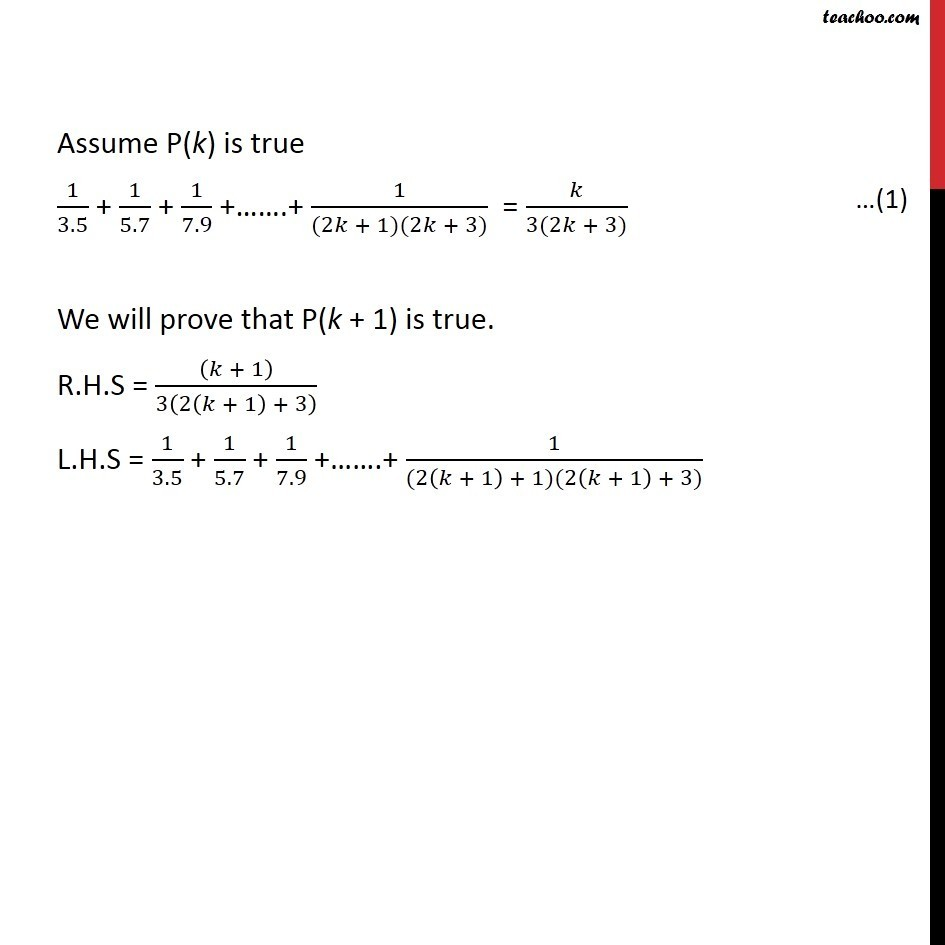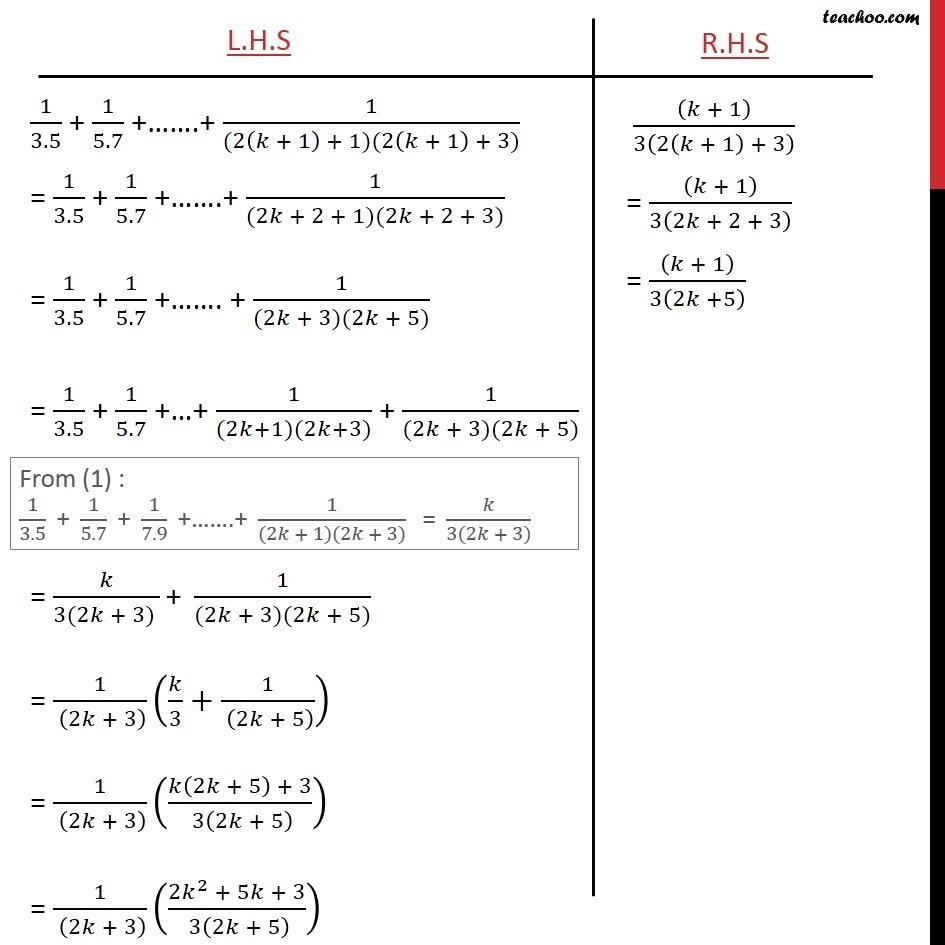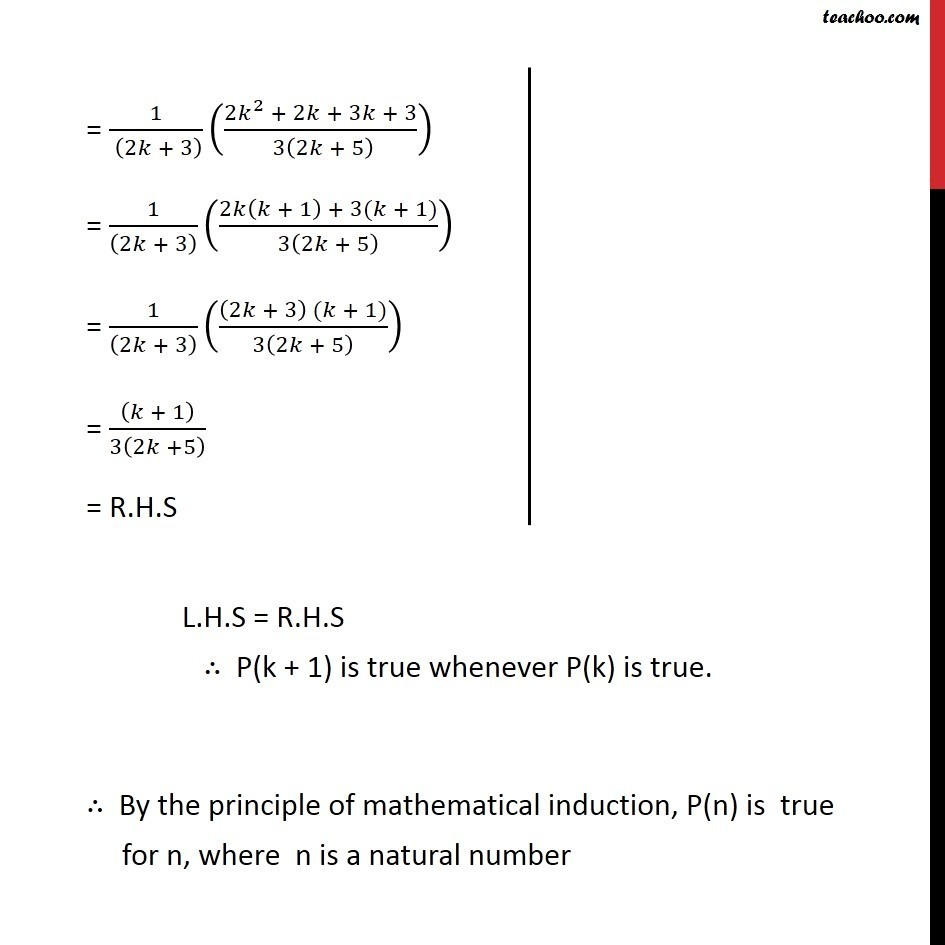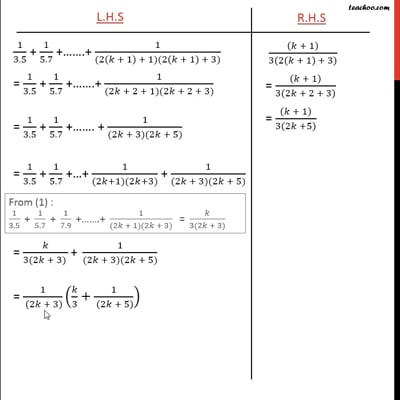This video is only available for Teachoo black users

Maths Crash Course - Live lectures + all videos + Real time Doubt solving!

### Transcript

Ex 4.1,17 Prove the following by using the principle of mathematical induction for all n N: 1/3.5 + 1/5.7 + 1/7.9 + .+ 1/((2 + 1)(2 + 3)) = /(3(2 + 3)) Let P (n) : 1/3.5 + 1/5.7 + 1/7.9 + .+ 1/((2 + 1)(2 + 3)) = /(3(2 + 3)) For n = 1, L.H.S = 1/3.5 = 1/15 R.H.S = 1/(3(2(1) + 3)) = 1/(3(2 +3)) = 1/(3 5) = 1/15 Hence, L.H.S. = R.H.S , P(n) is true for n = 1 Assume P(k) is true 1/3.5 + 1/5.7 + 1/7.9 + .+ 1/((2 + 1)(2 + 3)) = /(3(2 + 3)) We will prove that P(k + 1) is true. R.H.S = (( + 1))/3(2( + 1) + 3) L.H.S = 1/3.5 + 1/5.7 + 1/7.9 + .+ 1/((2( + 1) + 1)(2( + 1) + 3))